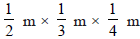Mensuration - MCQ

# Mensuration - MCQ

Test Description

## 10 Questions MCQ Test Class 8 Mathematics by VP Classes | Mensuration - MCQ

Mensuration - MCQ for Class 8 2022 is part of Class 8 Mathematics by VP Classes preparation. The Mensuration - MCQ questions and answers have been prepared according to the Class 8 exam syllabus.The Mensuration - MCQ MCQs are made for Class 8 2022 Exam. Find important definitions, questions, notes, meanings, examples, exercises, MCQs and online tests for Mensuration - MCQ below.
Solutions of Mensuration - MCQ questions in English are available as part of our Class 8 Mathematics by VP Classes for Class 8 & Mensuration - MCQ solutions in Hindi for Class 8 Mathematics by VP Classes course. Download more important topics, notes, lectures and mock test series for Class 8 Exam by signing up for free. Attempt Mensuration - MCQ | 10 questions in 10 minutes | Mock test for Class 8 preparation | Free important questions MCQ to study Class 8 Mathematics by VP Classes for Class 8 Exam | Download free PDF with solutions
 1 Crore+ students have signed up on EduRev. Have you?
Mensuration - MCQ - Question 1

### Which of the following is the once of a rhombus?

Mensuration - MCQ - Question 2

### If the edge of a cube is 1cm then which of the following is its volume?

Mensuration - MCQ - Question 3

### If the parallel sides of a parallelogram are 2 cm apart and their sum is 10 cm then its area is:

Detailed Solution for Mensuration - MCQ - Question 3

Distance between the two parallel lines = h =2 cm
sum of length of sides =10cm
As we know the sides of parallelogram are equal
let the length of side be 'b' =base of parallelogram
2b =10
b = 5 cm
area of parallelogram = h×b
= 2×5
=10 cm2

Mensuration - MCQ - Question 4

Which of the following has its area and perimeter numerically equal?

Mensuration - MCQ - Question 5

If the edge of a cube is 1 cm then which of the following is its total surface area?

Mensuration - MCQ - Question 6

Which of the following is equal to 1 kilolitre?

Mensuration - MCQ - Question 7

If the dimensions of a room are l, b and h, (∴ l → length, l → breadth and h → hight) themwhich of the following is the area of its four walls?

Mensuration - MCQ - Question 8

If the dimensions of a room are 2 m, 3 and 4 m then which of the following is the number ofcubes of sizewhich can he placed is the room?

Mensuration - MCQ - Question 9

If base area of a room 12 m2 and height is 3 m then its volume is:

Mensuration - MCQ - Question 10

Two identical cubes each of total surface area of 6 cm2 are joined end to end. Which of thefollowing is the total surface area of the cuboid so formed?

## Class 8 Mathematics by VP Classes

90 docs|16 tests
 Use Code STAYHOME200 and get INR 200 additional OFF Use Coupon Code
Information about Mensuration - MCQ Page
In this test you can find the Exam questions for Mensuration - MCQ solved & explained in the simplest way possible. Besides giving Questions and answers for Mensuration - MCQ, EduRev gives you an ample number of Online tests for practice

90 docs|16 tests